# 简单回顾卷积¶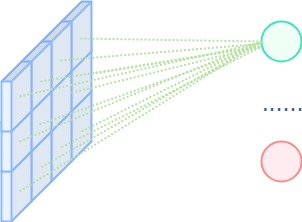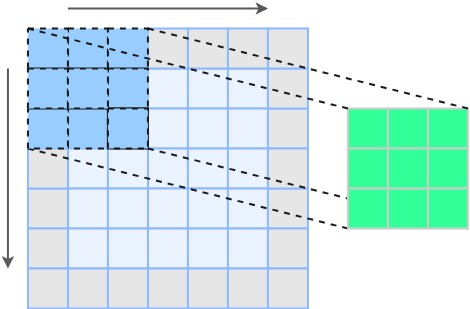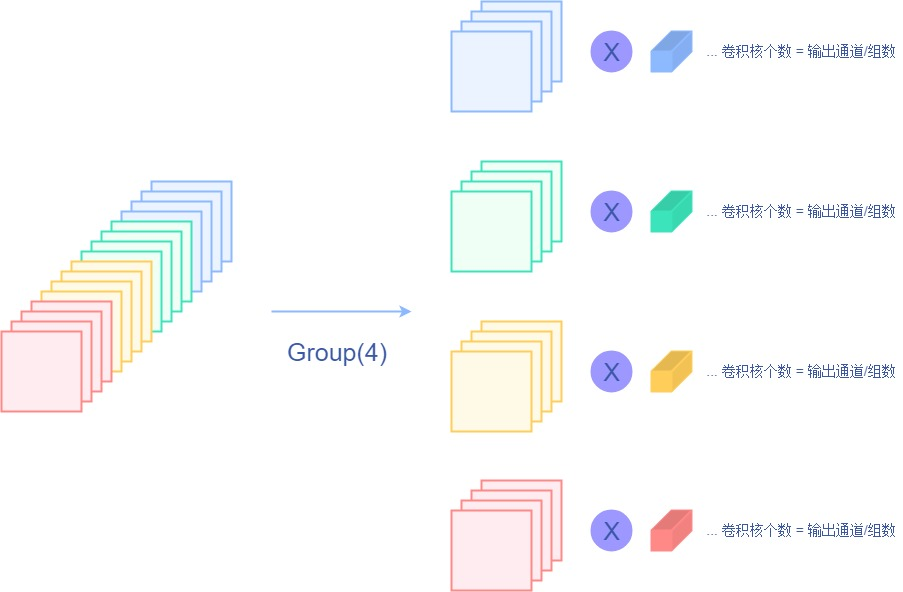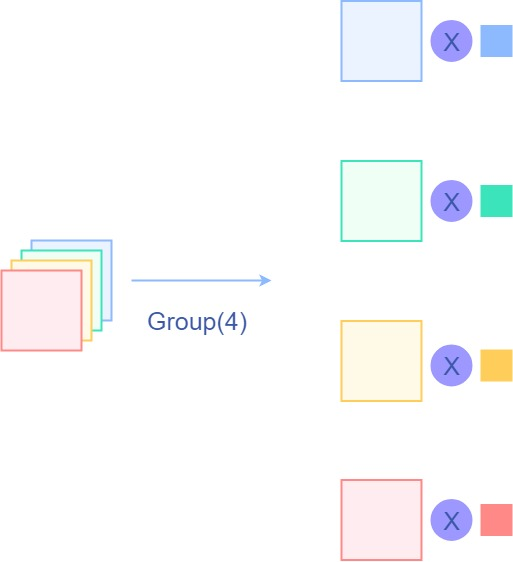# Involution的设计原则¶

Involution的设计原则就是颠倒常规卷积核的两个设计原则，即从空间无关性，频域特殊性转变成空间特殊性频域无关性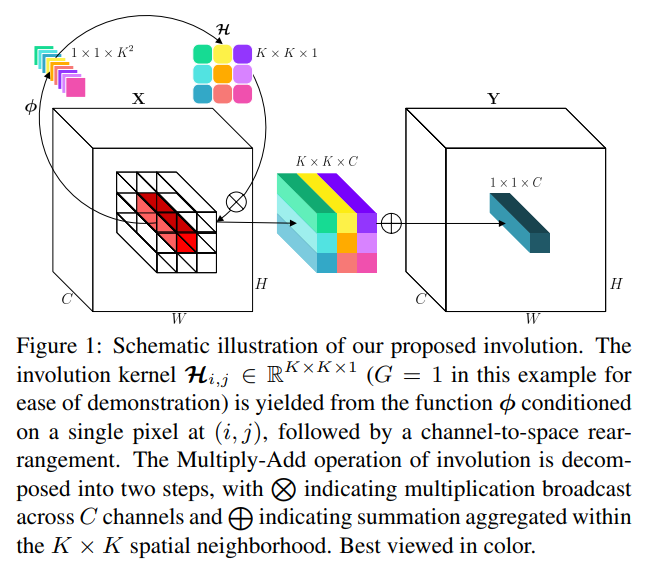H_{i, j} = \phi(X_i, j) = W_1\sigma(W_0(X_{i, j}))

    ...
reduction_ratio = 4
self.group_channels = 16
self.groups = self.channels // self.group_channels
self.conv1 = ConvModule(
in_channels=channels,
out_channels=channels // reduction_ratio,
kernel_size=1,
conv_cfg=None,
norm_cfg=dict(type='BN'),
act_cfg=dict(type='ReLU'))
self.conv2 = ConvModule(
in_channels=channels // reduction_ratio,
out_channels=kernel_size**2 * self.groups,
kernel_size=1,
stride=1,
conv_cfg=None,
norm_cfg=None,
act_cfg=None)
def forward(self, x):
weight = self.conv2(self.conv1(x if self.stride == 1 else self.avgpool(x)))
...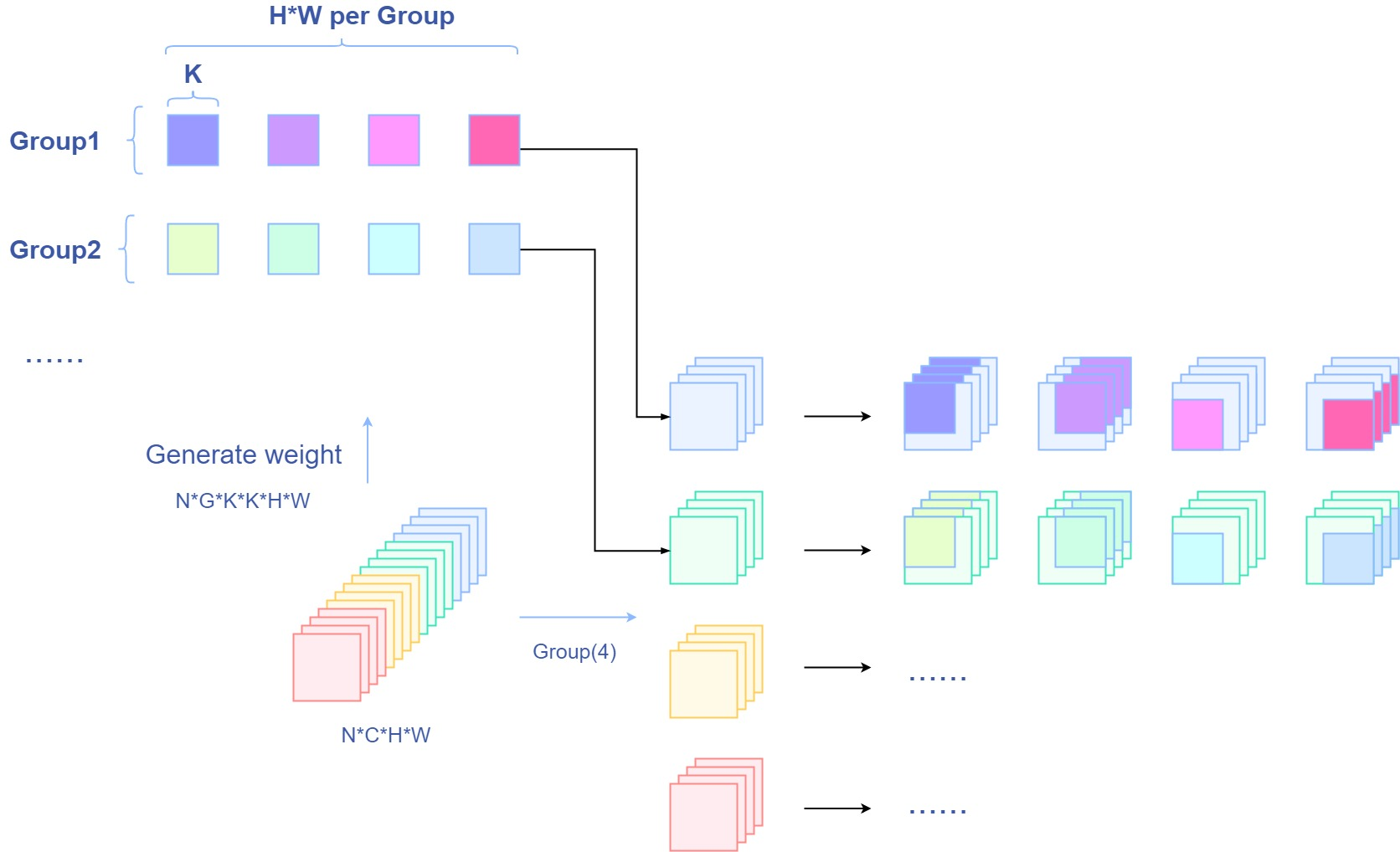import torch.nn as nn
from mmcv.cnn import ConvModule

class involution(nn.Module):

def __init__(self,
channels,
kernel_size,
stride):
super(involution, self).__init__()
self.kernel_size = kernel_size
self.stride = stride
self.channels = channels
reduction_ratio = 4
self.group_channels = 16
self.groups = self.channels // self.group_channels
self.conv1 = ConvModule(
in_channels=channels,
out_channels=channels // reduction_ratio, # 通过reduction_ratio控制参数量
kernel_size=1,
conv_cfg=None,
norm_cfg=dict(type='BN'),
act_cfg=dict(type='ReLU'))
self.conv2 = ConvModule(
in_channels=channels // reduction_ratio,
out_channels=kernel_size**2 * self.groups,
kernel_size=1,
stride=1,
conv_cfg=None,
norm_cfg=None,
act_cfg=None)
if stride > 1:
# 如果步长大于1，则加入一个平均池化
self.avgpool = nn.AvgPool2d(stride, stride)
self.unfold = nn.Unfold(kernel_size, 1, (kernel_size-1)//2, stride)

def forward(self, x):
weight = self.conv2(self.conv1(x if self.stride == 1 else self.avgpool(x))) # 得到involution所需权重
b, c, h, w = weight.shape
weight = weight.view(b, self.groups, self.kernel_size**2, h, w).unsqueeze(2) # 将权重reshape成 (B, Groups, 1, kernelsize*kernelsize, h, w)
out = self.unfold(x).view(b, self.groups, self.group_channels, self.kernel_size**2, h, w) # 将输入reshape
out = (weight * out).sum(dim=3).view(b, self.channels, h, w) # 求和，reshape回NCHW形式
return out


# 实验结果¶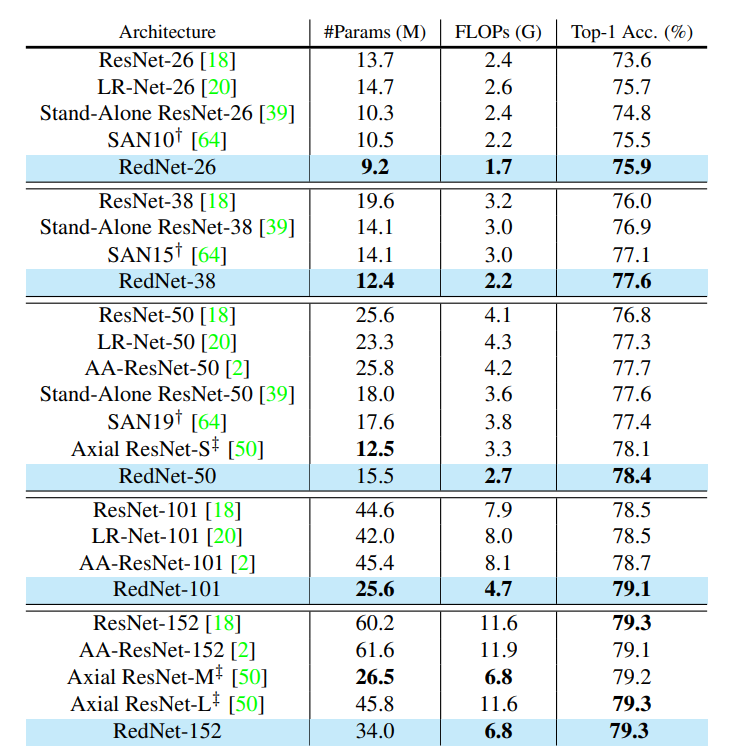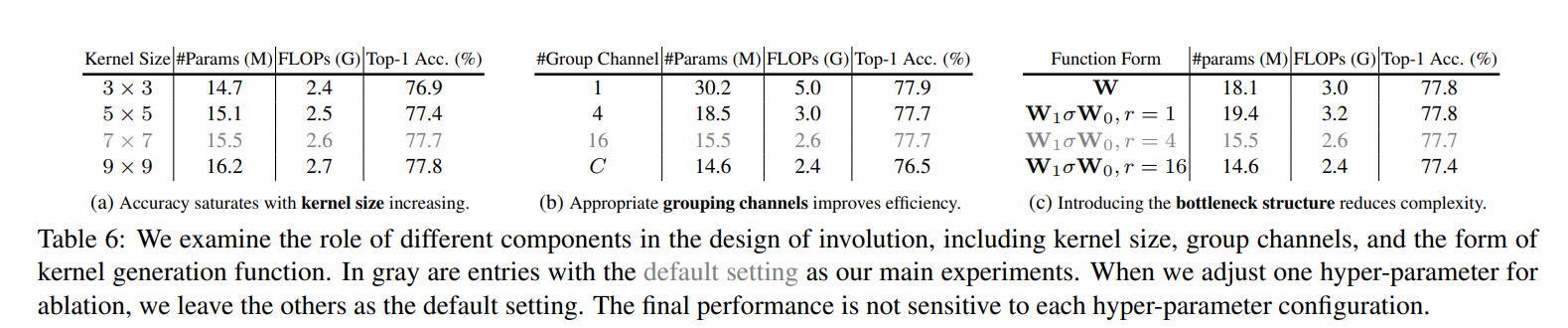# 总结¶